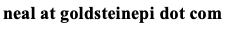Neal D. Goldstein, PhD, MBI

Dec 5, 2018

Relationships between observed outcomes and true prevalences

Editor's Note: I would like to welcome Daniel Vader, Department of Epidemiology and Biostatistics at the Drexel University Dornsife School of Public Health, as a guest blogger. The next couple of posts will demonstrate report generation within R using Sweave, something which I am very interested in and has broad applications including dynamic table creation for academic articles, as well as the articles themselves. This post serves as the didactic example that Dan created; the next post will be a presentation of the R code used to create it. Although this report was generated as PDF file, I am presenting it here as a web page for convenience. Dan can be contacted at dtv28 at drexel dot edu. My sincere thanks for an excellent contribution to this blog, Dan.

1. Introduction

Whenever I introduce the topic of misclassification to a class, I will invariably show students a 2x2 test validity table with the true classification of a condition in the columns and the test-based classification in the rows (Table 1). Since my lesson on misclassification comes after I introduce students to the basics of probability, I present measures of validity -- sensitivity (Se), specificity (Sp), positive predictive value (PPV), and negative predictive value (NPV) -- in terms of conditional probability. We conclude by discussing how wildly positive predictive value can change depending on the true prevalence of the outcome, hammering home some of the epistemological consequences of misclassification.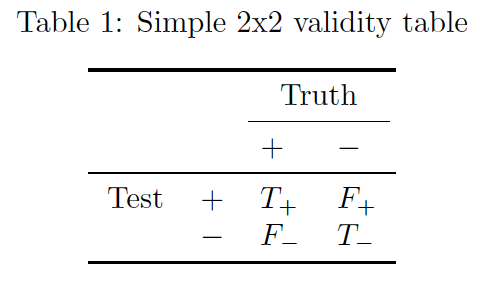Perhaps this lesson sounds familiar. I have seen variants of it (usually excluding discussion of conditional probability) in courses that I took as a masters student, in graduate and undergraduate courses where I was a teaching assistant, and finally in the materials left to me by the prior instructor of a graduate-level introduction to epidemiology and biostatistics course that I have now taught for three years. Perhaps the lesson is so prevalent in my academic circle because, at its core, it is a good lesson, an easy gateway to the beautifully tangled world of measurement error where example data are neatly formatted in a small table and probabilities can be described using simple proportions.

However, while I continue to believe that this approach serves as good introduction to the basic concepts of misclassification, what may limit more curious students is that my discussion of the relationship between measures of validity is fairly superficial. I, or another teacher, may communicate the hard and fast rule that "When true prevalence decreases, PPV also decreases," but we may not directly give students the tools to understand the relationship between parameters more thoroughly ("Here is how we can predict PPV based on sensitivity, specificity, and prevalence").

This article is intended to be a bridge between very basic lessons on misclassification and relational understanding of observed prevalence (P*), positive predictive value, true prevalence (P), sensitivity, and specificity. The article assumes that your students have already had a lesson in basic probability, including the rule of subtraction, Pr(A)=1-Pr(A'), and the rule of multiplication, Pr(A ∩ B)=Pr(A)Pr(B|A). I understand that, when a particular cohort has vocal phobia of basic algebra, avoiding discussion of the relationship between PPV or P* and P, Se, and Sp is a tempting shortcut. But we should be prepared to expect more of our students on this topic, or at the very least have materials at hand to give questioning students the tools they need to satisfy their curiosity.

2. Deriving and describing relationships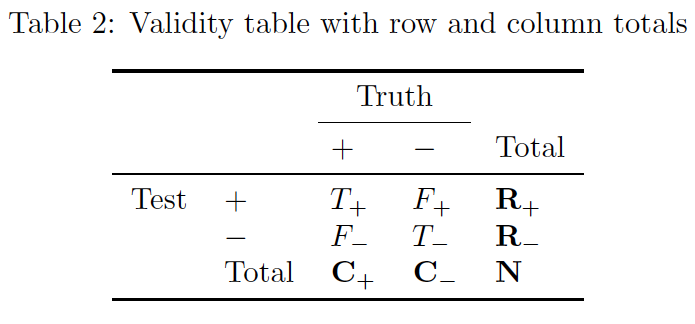Let us begin by extending table 1 so that we have the notation to more simply discuss the marginal probabilities (table 2). With notation for the row and column totals established, we can now prescribe meaning to each of the cells in the table. You may already be familiar with a similar notation for defining measures of validity, but sometimes a refresher can be useful:

• T+ = true positives, F- = false negatives, T+ + F- = C+ = all subjects who actually have the condition
• F+ = false positives, T- = true negatives, F+ + T- = C- = all subjects who actually do not have the condition
• R+ and R- represent the total number of subjects who tested positive and tested negative respectively.
• N = the total number of subjects.

Next, let us define prevalence (P), observed prevalence (P*), sensitivity(Se), specificity (Sp), and positive predictive value (PPV) using both proportions and probabilities. If you learned about or best remember these measures using proportions, take a moment to familiarize yourself with the probabilities and what they mean.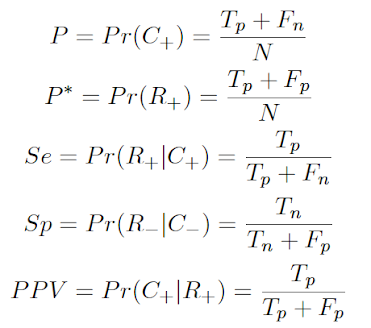2.1 Positive predictive value

By revisiting the rule of multiplication, Pr(A ∩ B)=Pr(A)Pr(B|A), and the rule of subtraction, Pr(A)=1-Pr(A'), we can rewrite the component parts of PPV in terms of P, Se and Sp:With a formula in hand, we can now begin to make observations about the relationship between PPV and P, Se, and Sp. I find that visualizing this relationship helps both my students and myself to internalize its operation. To this end, a straightforward approach is to write a basic function that calculates PPV using the described formula:

> ppv.calc <- function(p, se, sp){
+ ppv <- (p*se)/(p*se+(1-p)*(1-sp))
+ return(ppv)
+ }

The ppv.calc function allows us to estimate PPV simply by inputting values for P, Se, and Sp. While setting these numbers individually may be helpful in circumstances where we have a very good idea how to define our parameters, examining a range of values is more useful for a general description of the relationship between those parameters and PPV (Figure 1).On Figure 1 we see much greater variation by Sp (color) than by Se (line style) across values of prevalence. When Sp is high, the PPV curve is more favorable than when Sp is low. On the other hand, the PPV curve is only marginally more favorable for higher values of Se. Furthermore, notice how PPV approaches 0 in all scenarios as prevalence approaches 0. Likewise, note how PPV always approaches 1 as prevalence approaches 1.

The bones of these observations are also evident in the PPV equation that we derived. Se is equally weighted by P in both the numerator and denominator of the equation, meaning that these two pieces of the fraction tend to balance each other out. On the other hand, Sp is weighted by the inverse of P and is only present in the denominator. Thus, as P decreases, the weight of Sp in the denominator increases while simultaneously the weight of Se decreases.

2.2 Observed prevalence

Using the same substitution approach employed to describe PPV, we can also rewrite the equation for observed prevalence (P*):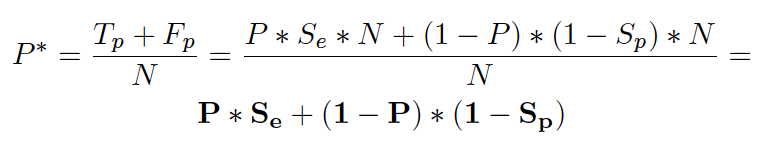Since Se is no longer balanced on the numerator and denominator of the equation, we know immediately that Se plays a more important role when determining P* than when determining PPV. To examine these relationships more closely, let us again store the equation as a function and graph that function.

> prev.calc <- function(p, se, sp){
+ op <- p*se + (1-p)*(1-sp)
+ return(op)
+ }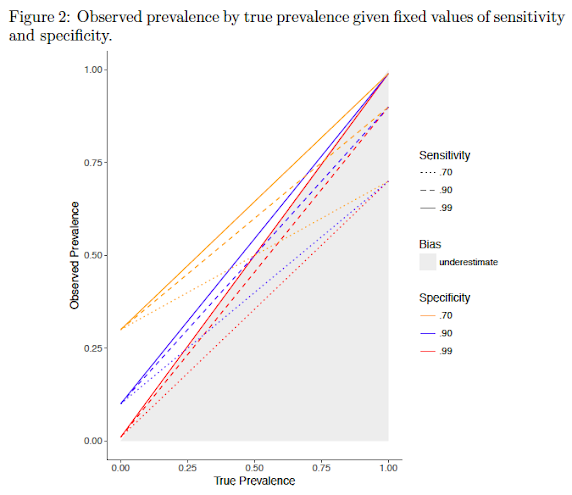The pattern in Figure 2 is a little more difficult to see at first glance compared to the pattern in Figure 1, but becomes clear once you spend a little time with it. Observe how lines of the same color converge as P approaches 0 and diverge as P approaches 1. Since line style represents levels of sensitivity and line color represents levels of specificity, we can say that observed prevalence becomes more dependent on sensitivity as true prevalence increases. Likewise, observing that lines of the same style converge as P approaches 1 and diverge as it approaches 0, we know that observed prevalence becomes more dependent on specificity as true prevalence decreases.

As with our observations on PPV, the patterns we see in Figure 2 are also seen in the equation that we derived for P*. Se is weighted by P and Sp is weighted by 1-P. Thus, the shifting importance of Se and Sp with P is to be expected.

What is perhaps less intuitive is how the bias associated with P* as an estimator of P changes with different levels of Se, Sp, and P. When Se is very high, P* is more likely to overestimate P, particularly when P is low. This is due to the fact that few subjects who are positive for the condition of interest are being classified as negative when Se is very high, but there is still potential for subjects without the condition to test postive if Sp is not also very high. Likewise, when Sp is very high, P* is more likely to underestimate P, particularly when P is high. When both Se and Sp are equal and not near perfect, P becomes the dominant indicator of over or underestimation.

For those of us who like to see tables filled with numbers in addition to graphs, Table 3 shows how observed prevalence (P*) changes depending on values of P, Se, and Sp. The P*-P column represents the degree to which observed prevalence over or under-estimates true prevalence in each scenario. Tables like these may be useful when discussing specific examples, Figures 1 & 2 may be more useful when discussing the equations and the relationships the equations indicate.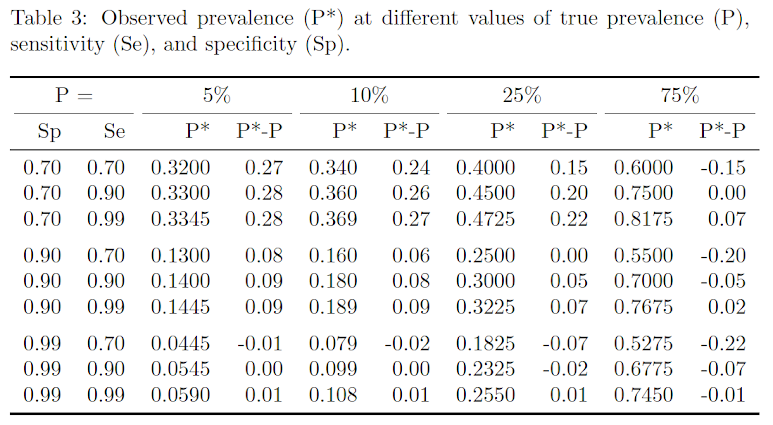3. Conclusion

Exploring the validity of screening test results in the classroom gives students an opportunity to engage with a number of important topics that are foundational to epidemiology (including misclassification and probability), and it is one of my favorite lessons to teach. This paper has presented resources for helping your students to move beyond a superficial understanding of the relationship between features of the test and observed outcome. Of particular note is our examination of observed prevalence which, in my experience, is often completely neglected.